﻿ 船载无人机惯性导航系统中的数据实时融合算法
 舰船科学技术2022, Vol. 44Issue (10): 131-134    DOI: 10.3404/j.issn.1672-7649.2022.10.027PDF

1. 河南理工大学 鹤壁工程技术学院，河南 鹤壁 458030;
2. 鹤壁职业技术学院，河南 鹤壁 458030

Data real-time fusion algorithm in ship borne UAV inertial navigation system
ZHENG Jin-song1,2, GU Hai-hong1,2, JIANG Qing-gang1,2
1. Hebi Institute of Engineering and Technology, Henan Polytechnic University, Hebi 458030, China;
2. Hebi Polytechnic, Hebi 458030, China
Abstract: The inertial navigation system of ship borne UAV integrates inertial components such as accelerometer, gyroscope and GPS. It is an indispensable system for the positioning, navigation and landing control of ship borne UAV. This paper focuses on the multi-sensor data real-time fusion algorithm of ship borne UAV inertial navigation system, and analyzes the overall working principle of inertial navigation system, including system components, coordinate conversion principle The attitude calculation of UAV and the data fusion of time and space position are analyzed in detail. The research of this paper is of great significance to improve the navigation accuracy of ship borne UAV.
Key words: ship borne UAV     inertial navigation system     multi sensor     data fusion     attitude solution
0 引　言

1 船载无人机惯性导航系统的数据融合需求

1）陀螺仪等传感器直接安装在船载无人机上，采集无人机的加速度和旋转速率等信息；

2）通过数学矩阵的方法进行无人机位置、速度等信息的推算，陀螺仪和加速度计采集一定坐标系下的船载无人机数据，然后经过坐标转换和数学推算，获取当前导航坐标系下正确的目标分量。

3）姿态矩阵是船载无人机SINS系统的核心，计算机的强大性能保证数学矩阵的解算效率。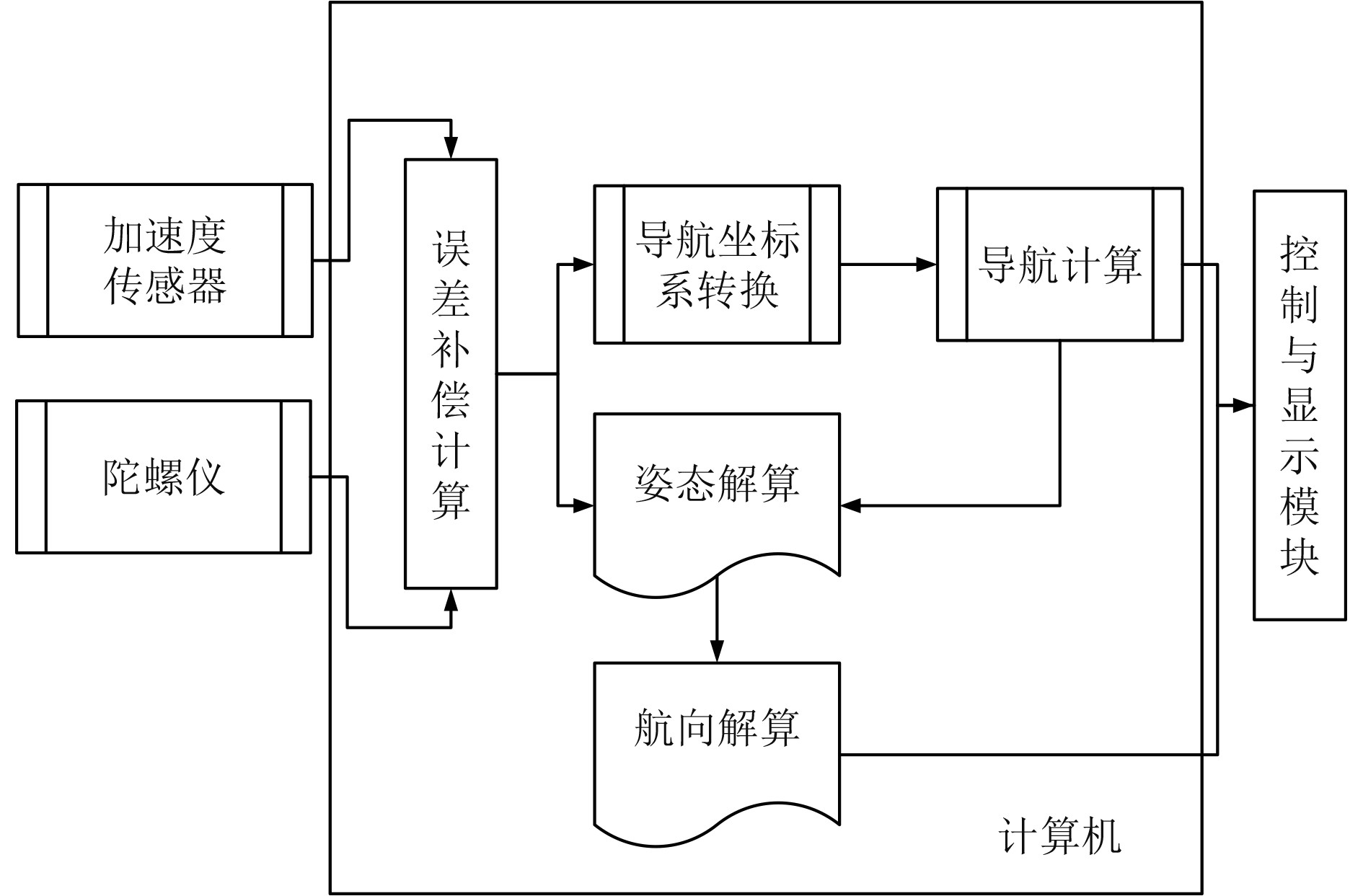图 1 船载无人机捷联惯性导航系统工作原理图 Fig. 1 Working principle diagram of shipborne UAV strapdown inertial navigation system

2 船载无人机惯性导航系统的坐标转换和关键部件 2.1 船载无人机惯性导航系统的坐标转换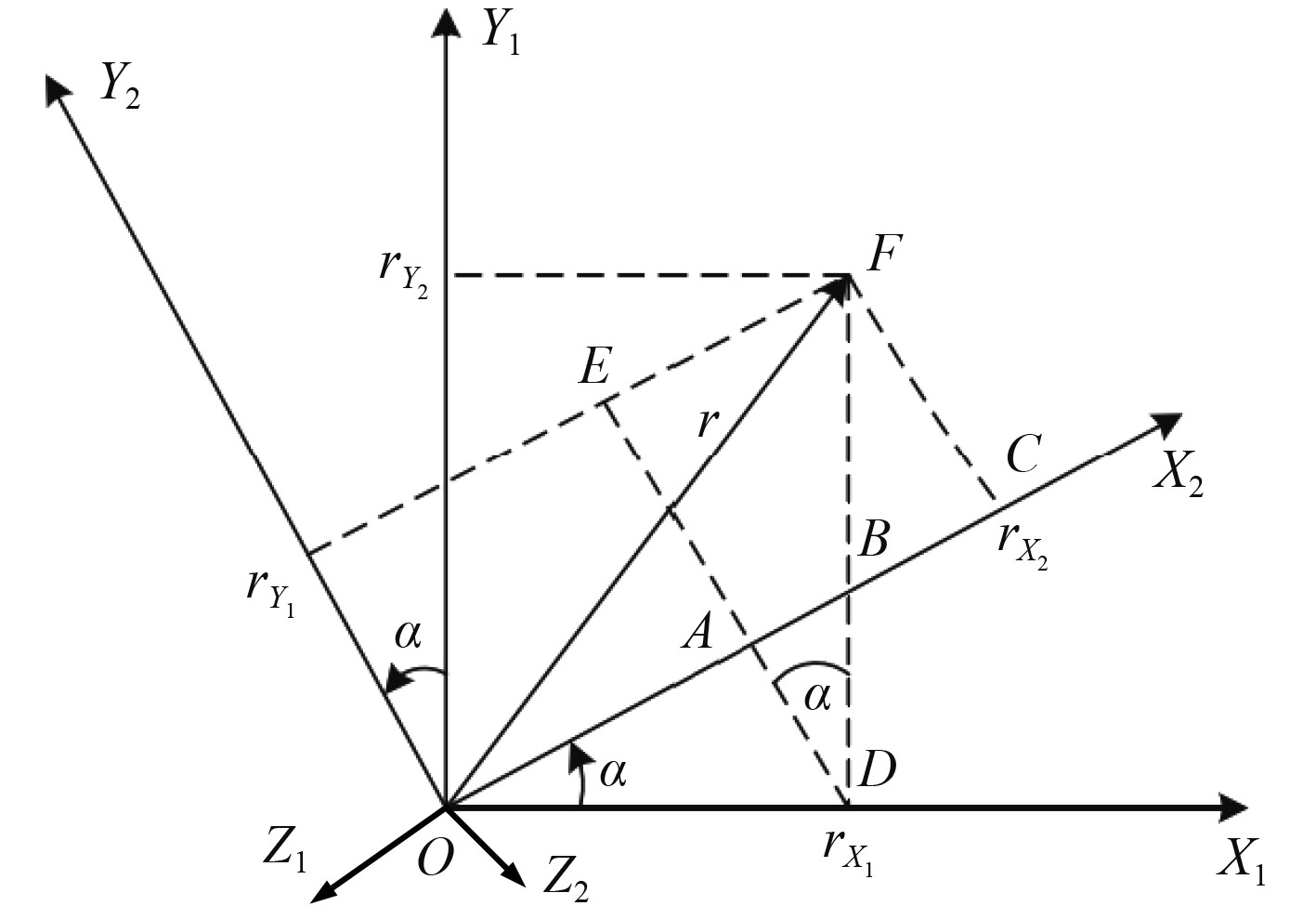图 2 船载无人机惯性导航系统的坐标系 Fig. 2 Coordinate system of inertial navigation system of ship borne UAV

 $\left[ {\begin{array}{*{20}{c}} {{r_{{x_2}}}} \\ {{r_{{y_2}}}} \\ {{r_{{Z_2}}}} \end{array}} \right] = \left[ {\begin{array}{*{20}{c}} {\cos \alpha }&{\sin \alpha }&0 \\ { - \sin \alpha }&{\cos \alpha }&0 \\ 0&0&1 \end{array}} \right]\left[ {\begin{array}{*{20}{c}} {{r_{{x_1}}}} \\ {{r_{{y_1}}}} \\ {{r_{{z_1}}}} \end{array}} \right] \text{，}$

 $O - {X_1}{Y_1}{Z_1} \to O - {X_2}{Y_2}{Z_2} \to O - {X_n}{Y_n}{Z_n} \text{，}$

 ${\boldsymbol{M}}_1^2 = \left[ {\begin{array}{*{20}{c}} 1&0&0 \\ 0&{\cos \theta }&{\sin \theta } \\ 0&{ - \sin \theta }&{\cos \theta } \end{array}} \right] \text{，} {\boldsymbol{M}}_n^1 = \left[ {\begin{array}{*{20}{c}} {\cos \psi }&{ - \sin \psi }&0 \\ {\sin \psi }&{\cos \psi }&0 \\ 0&0&1 \end{array}} \right] \text{，}$

 ${\boldsymbol{M}}_n^b = {\boldsymbol{M}}_1^2{\boldsymbol{M}}_n^1 = \left[ {\begin{array}{*{20}{c}} {\cos \gamma }&0&{ - \sin \gamma } \\ 0&1&0 \\ {\sin \gamma }&0&{\cos \gamma } \end{array}} \right]\left[ {\begin{array}{*{20}{c}} {\cos \psi }&{ - \sin \psi }&0 \\ {\sin \psi }&{\cos \psi }&0 \\ 0&0&1 \end{array}} \right] 。$
2.2 船载无人机惯性导航系统关键部件

1）惯性导航组件

2）GPS

GPS可以为船载无人机提供全天候、高精度的定位信息，目前，GPS技术已经具备更高的集成特性，模块体积更小，性能更强。船载无人机惯导系统使用GPS进行三维定位、测速、授时等功能。

3）多普勒测速仪DVL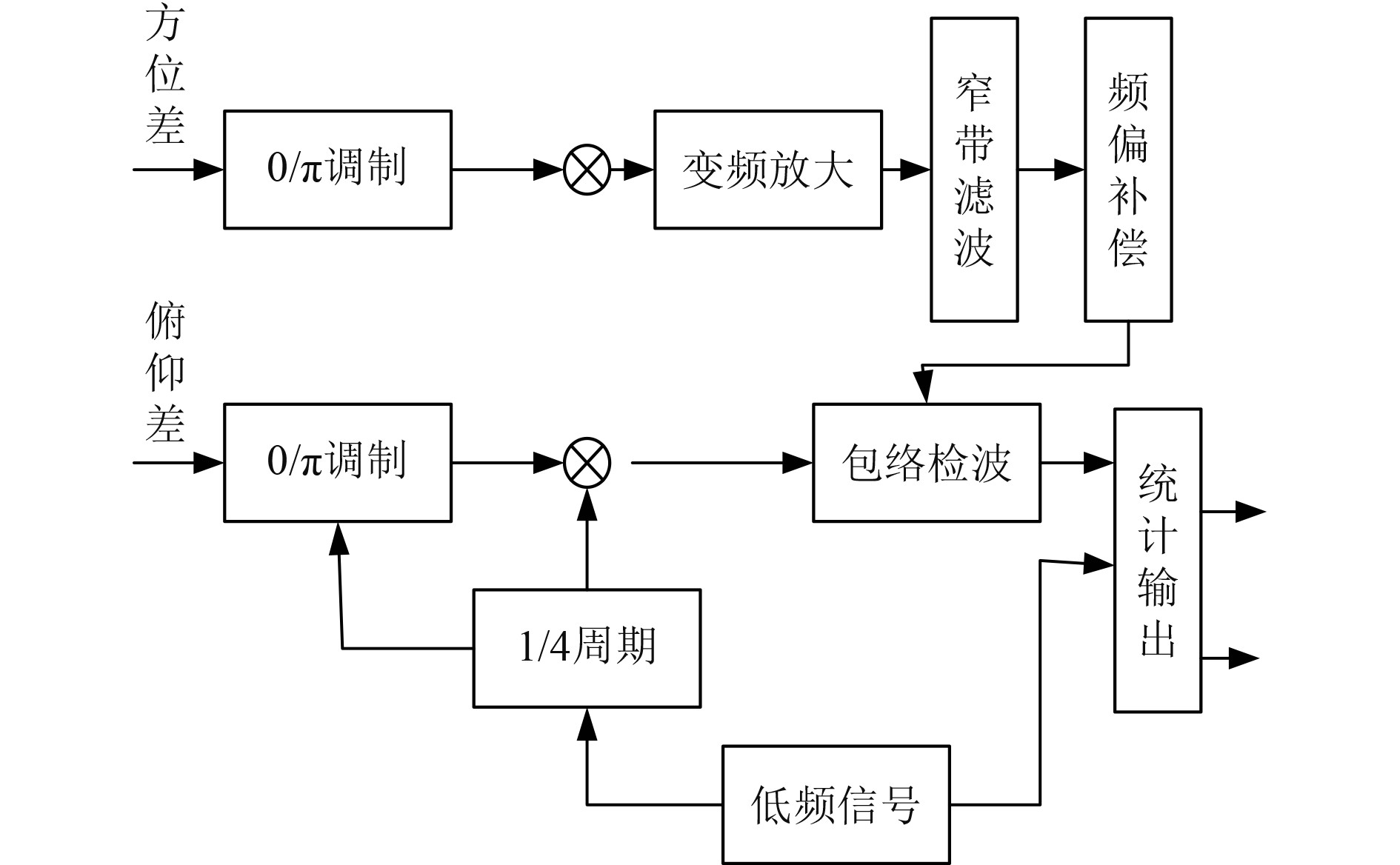图 3 多普勒测速仪DVL的测速原理 Fig. 3 Velocity measurement principle of Doppler velocimeter DVL

 ${f^\prime } = \frac{{{v_0}}}{v}f 。$

 ${f^{\prime \prime }} = \frac{{v + {v_0}}}{v}{f^\prime } = \frac{{v + {v_0}}}{{v - {v_0}}}f \text{，}$

 ${f_d} = {f^{\prime \prime }} - f = \frac{{2{v_0}}}{{v - {v_0}}}f \text{，}$

 $\begin{gathered} v = \left( {1 + \frac{{2f}}{{{f_d}}}} \right){v_0} \text{，} \\ {v_0} = \left( {\frac{{{f_d}}}{{2f + {f_d}}}} \right)v \text{。} \\ \end{gathered}$
3 船载无人机惯性导航系统的数据融合 3.1 惯性导航系统的异步传感器采样

 $S_i = \frac{{{S_N}}}{{{n_i}}} \text{，}$

 $\begin{gathered} x(N,k + 1) = {\boldsymbol{\varPhi}} (N,k)x(N,k) + w(N,k) ，\\ z(i,k) = {\boldsymbol{H}}(i,k)x(i,k) + v(i,k) 。\\ \end{gathered}$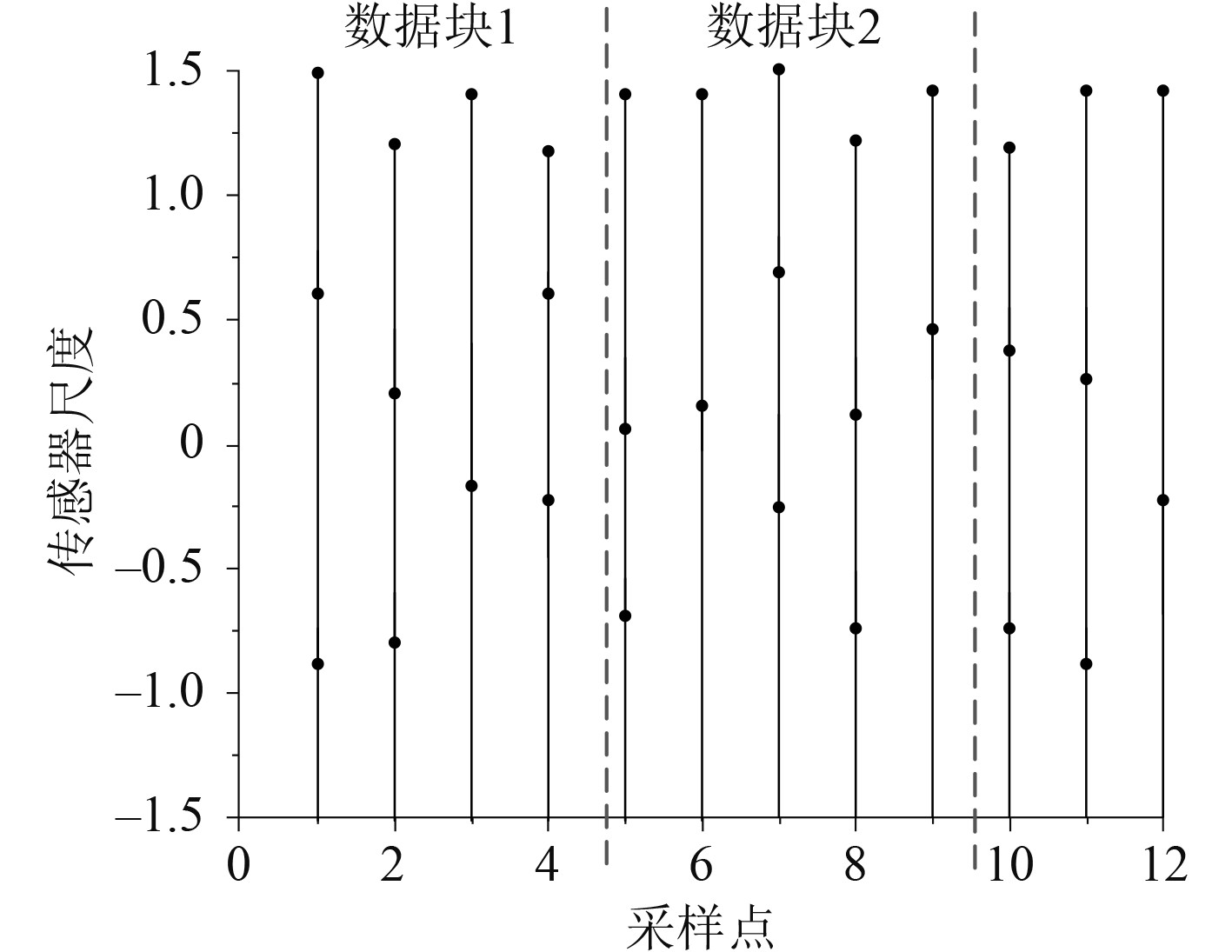图 4 不同尺度的传感器采样点与时刻的关系图 Fig. 4 Relationship between sampling points and time of sensors with different scales
3.2 惯性导航系统的多传感器信号融合算法

1）船载无人机姿态信号融合

 $\left\{ \begin{gathered} x = \rho \sin {\theta _t} ，\\ y = \rho \cos {\theta _t} ，\\ \end{gathered} \right.$

 $\begin{gathered} {\sigma _l} = {\sigma _0}\cos {\theta _t} + \rho \sin {\theta _t} ，\\ {\sigma _s} = {\sigma _0}\sin {\theta _t} + \rho \cos {\theta _t} ，\\ \end{gathered}$

 ${\left( {{x_0},{y_0},{z_0}} \right)^{\rm{T}}} = \left[ \begin{gathered} \cos {\theta _t} \hfill \\ 1 \hfill \\ \sin {\theta _t} \hfill \\ \end{gathered} \right] \times {\left( {\rho ,{\theta _t}} \right)^{\rm{T}}} \times \left[ \begin{gathered} {\sigma _l} \hfill \\ {\sigma _s} \hfill \\ \end{gathered} \right] 。$

2）船载无人机多传感器信号的时间关联

 $\left| {{k_{A,j}} - {k_{B,j}}} \right| < C\;\;,j = 0,1,\cdots ,n \text{，}$

 $\left\{ \begin{gathered} x\left( k \right) = x\left( {{t_i}} \right) + \frac{{\sin \left( {S\left( {{t_i}} \right) \cdot \left( {k - {t_i}} \right)} \right)}}{{60}} ，\\ y\left( k \right) = y\left( {{t_i}} \right) + \frac{{\cos \left( {S\left( {{t_i}} \right) \cdot \left( {k - {t_i}} \right)} \right)}}{{60}} 。\\ \end{gathered} \right.$

3）误差分析

 $S(x + 1) = \phi (x + 1,x)S(x) + H(x) \text{，}$

 $\mathop {\rm{S}}\limits^{\smallfrown} (x/x - 1) = \phi (x/x - 1)\mathop {\rm{S}}\limits^{\smallfrown} (x) ，$

 $\eta \left( {x/x} \right) = {\left[ {1 - S(x)C\left( x \right)P(x/x - 1)} \right]^{\frac{1}{2}}} 。$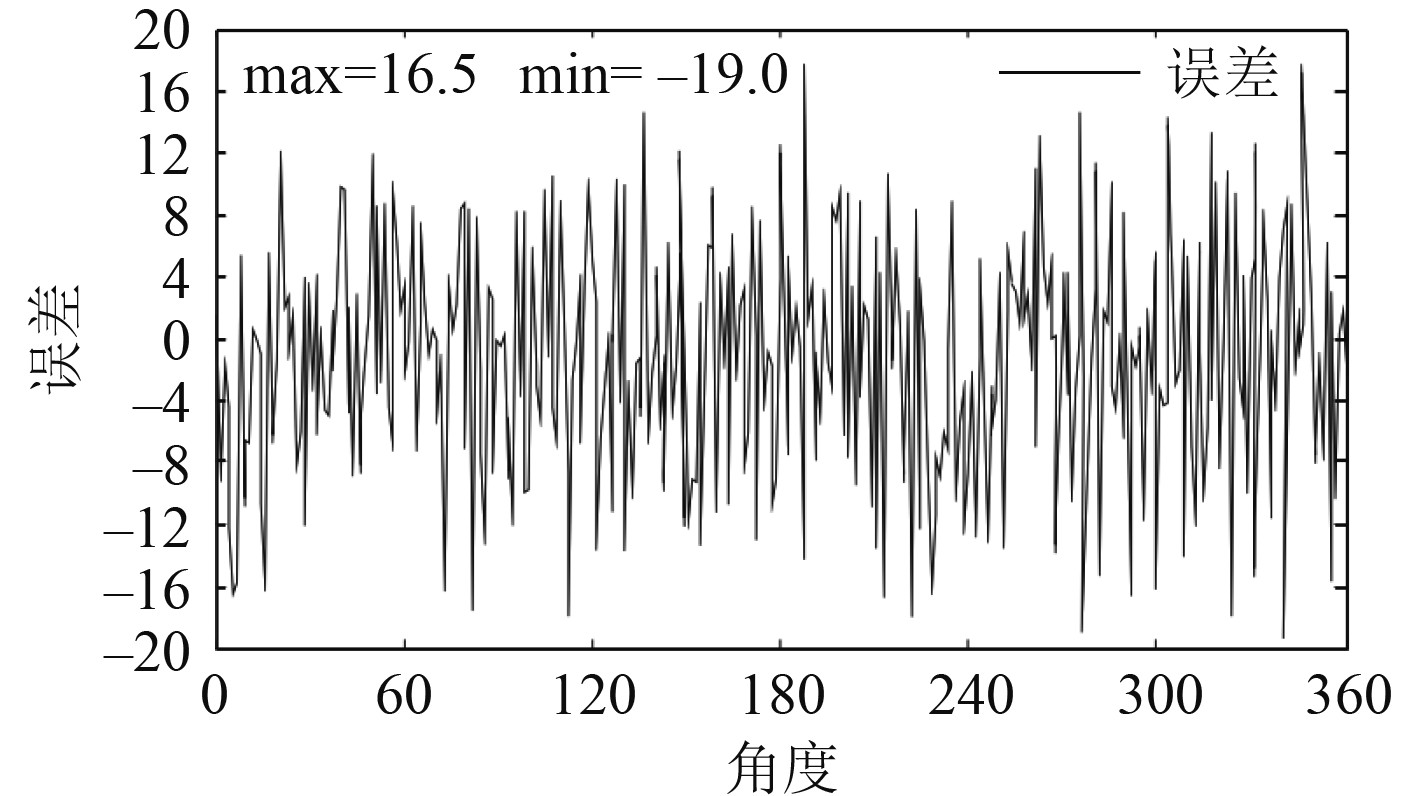图 5 0～360°无人机航行角度的信号融合误差曲线 Fig. 5 Signal fusion error curve of 0～360 ° UAV navigation angle
4 结　语

  杨功流, 李士心, 姜朝宇. 地磁辅助惯性导航系统的数据融合算法[J]. 中国惯性技术学报, 2007, 15(1): 4.  王勇军, 李智, 李翔. 无人机磁惯导系统中航向校正的双内积算法[J]. 仪器仪表学报, 2019, 40(9): 8.  刘卫华, 高贺, 高超. 适用于巡检无人机的捷联惯导系统快速对准研究[J]. 系统仿真技术, 2019(4): 5.  许多伦, 蔡宇哲, 薛松浩, 等. 基于惯导以及GPS的飞行器定位系统[J]. 信息记录材料, 2018, 19(11): 2.  王勇军, 李智, 李翔. 无人机磁惯导系统中陀螺仪的叉积标定算法[J]. 仪器仪表学报, 2020(4): 10.  卢鑫, 练军想, 吴美平. 高精度舰载惯性导航系统的重力影响研究[J]. 导航与控制, 2010, 9(4): 15-21.  罗续成. 惯性导航系统/双星距离差组合导航算法的可行性研究[J]. 遥测遥控, 2002(6): 7.